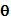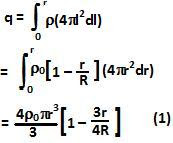## Wednesday, May 5, 2010

### Irodov Problem 3.25Since charge density is isotropic (does not depend onbut is only a function of only r), the electric field vector will be radially outwards at any point within or outside the sphere.

When r less than R

Let us consider a spherical Gaussian Surface that is concentric with the sphere and of radius r. Let us first compute the total charge contained within this Gaussian Surface. Consider an infinitesimally thin spherical shell of of radius l and thickness dl. The volume of this infinitesimally thin sphere will beand so the charge contained in this volume will be. We can compute the total charge in the Gaussian Sphere by integrating over all such infinitesimally thin spherical shells as,From Gauss Law we have,
The total charge contained in the Gaussian Surface is simply the total charge in the sphere is given by,
From Gauss Law we have,
The maximum value of Electric Field occurs when,